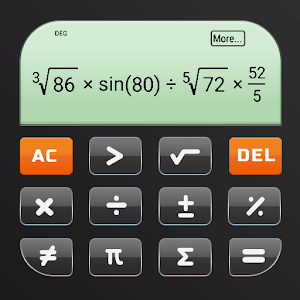English

Every day we offer FREE licensed Android apps and games you’d have to buy otherwise.3.99
EXPIRED

# Android Giveaway of the Day - Calculator+

A multi-funcional calculator.
3.99 EXPIRED
User rating: 0 (0%) 0 (0%)

This giveaway offer has expired. Calculator+ is now available on the regular basis.

Multifunction Calculator Pro - Calculator Plus is an app which is an advanced scientific calculator great for advanced calculations, especially for the students.

Convenient and intelligent input system enables you to perform even the most complex operations in a matter of seconds.

Choose between a basic calculator or scientific calculator with more than 30 scientific operations

Supports calculating percentages, square roots, powers, trigonometric functions, drawing 2D and 3D graphs, calculating with variables and constants.

If you need a level greater than the basics, try with this Multifunction Calculator Pro - Calculator Plus application. This is a fully integrated application from simple calculations to the professional "engineer" modes that are different for you. It is a multifunction calculator, support a lot of different calculations and in particular, it can generate graphs.

Multifunction Calculator Pro - Calculator Plus makes math simple and fun with a layout for fast and easy calculations includes both a standard and scientific calculator. Solve all of your everyday calculations, perform all the basic calculations you could ever need including addition, subtraction, division, multiplication, and percentages

Access additional features from the main screen of Multifunction Calculator Pro - Calculator Plus using gestures. Use backspace anytime to correct a simple mistake, instead of starting over. Keep track of your results using the memory buttons and history screen. For more complicated and advanced problems, use options like squaring, square root, per cent and the pi button

Features

✪ Calculations in clear, elegant type that's easy to read
✪ The results is calculated automatically
✪ Copy/paste with a single button press
✪ Personalize!
✪ The set theme you like
✪ Do calculations without switching between the apps!
✪ Calculate percentages, square roots, powers, trigonometric functions!
✪ Plot 2D and 3D graphs!
✪ Do calculations with variables and constants
✪ Integrate and differentiate
✪ Do calculations with fractions and simplify expressions
✪ Do calculations with complex numbers

⚾️ Multifunction Calculator Pro - Calculator Plus is also an exceptional school calculator. Multifunction Calculator Pro is the perfect calculator for teachers, students, and working professionals. If you quit the calculator and go do something else, it's all still there whenever you come back. You'll never need to type the same calculation twice again making it the perfect math calculator to take the best notes and solve any problem.

Firebird LLC

Tools

1.0.0

5.3M

Everyone

### Compatibility:

4.0 and up